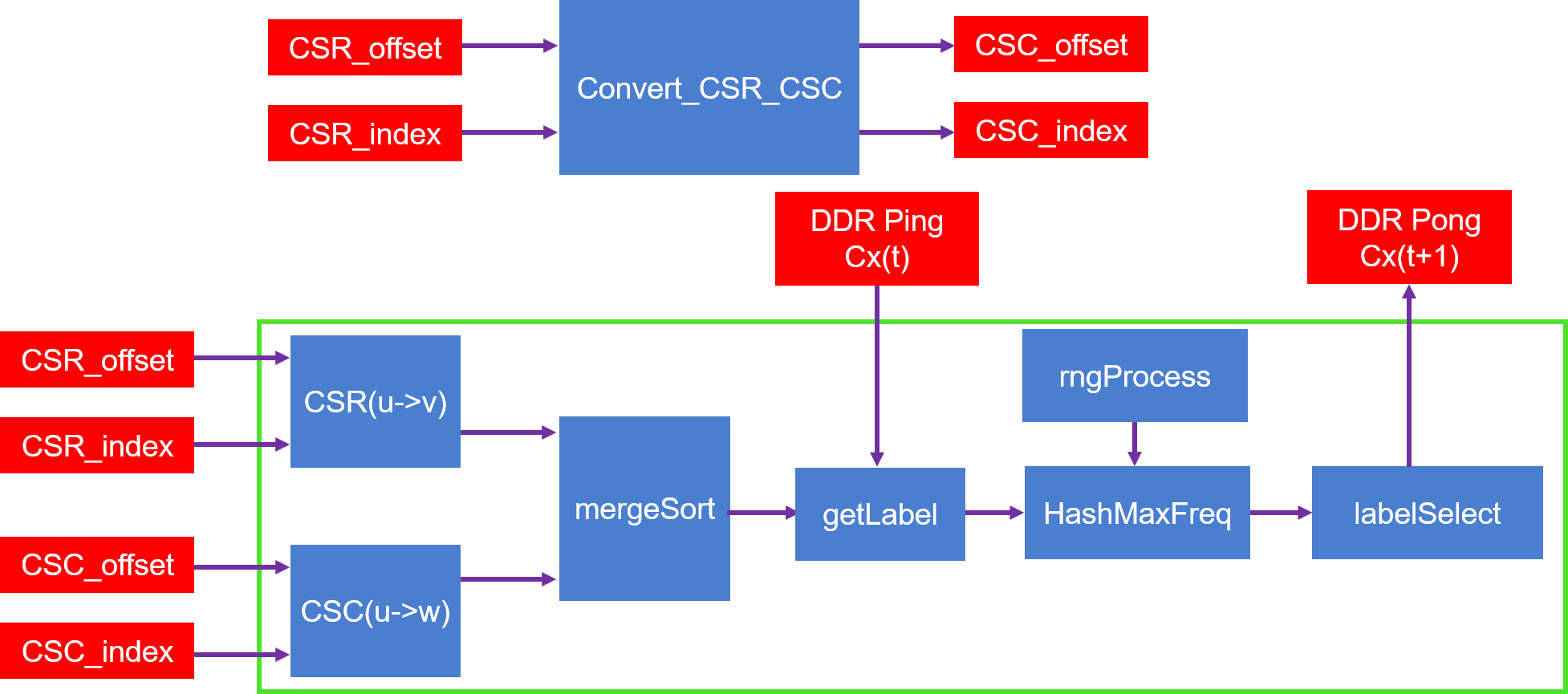# Internal Design of Label Propagation¶

## Overview¶

Within complex networks, real networks tend to have community structure. Label propagation is an algorithm for finding communities. In comparison with other algorithms label propagation has advantages in its running time and amount of a priori information needed about the network structure (no parameter is required to be known beforehand). The disadvantage is that it produces no unique solution, but an aggregate of many solutions.(from wikipedia)

## Algorithm¶

The algorithm of Label Propagation (synchronous update) is as follows:

1. Initialize the labels at all nodes in the network. For a given node $$x$$, $$C_{x}\left ( 0 \right )=x$$.
2. Set $$t = 1$$.
3. Arrange the nodes in the network in a random order and set it to $$X$$.
4. For each $$x\in X$$ chosen in that specific order, let $$C_{x}\left ( t \right )=f\left ( C_{x_{1}}\left ( t-1 \right )+\cdots +C_{x_{k}}\left ( t-1 \right )\right )$$. $$f$$ here returns the label occurring with the highest frequency among neighbors and ties are broken uniformly randomly.
5. If every node has a label that the maximum number of their neighbors have, then stop the algorithm. Else, set $$t = t + 1$$ and go to (3).

For more details, please see https://arxiv.org/abs/0709.2938

## Implemention¶

The algorithm implemention is shown as the figure below:As we can see from the figure:

1. Module Convert_CSR_CSC: convert compressed sparse row (CSR) format graph to compressed sparse column (CSC) format graph, When the iterNum is small, the module accounts for a large proportion of the total execution time of the LP_Kernel. the execution time of the module is reduced by increasing the cache depth.
2. Module getLabel and its previous module: get all labels of all neighbors of vertex u.
3. Module HashMaxFreq and labelSelect: find the highest frequency label (Select a label at random if there are multiple highest frequency labels), then output to DDR.

## Profiling¶

The hardware resource utilizations are listed in the following table.

 Kernel BRAM URAM DSP LUT Frequency(MHz) Platform 375 0 7 162080 LP_Kernel 100 0 0 72777 292

## Benchmark¶

The performance is shown in the table below.

 Datasets Vertex Edges FPGA time Spark (4 threads) Spark (8 threads) Spark (16 threads) Spark (32 threads) Spark time speedup Spark time speedup Spark time speedup Spark time speedup as-Skitter 1694616 11094209 27.85 1336.85 48.01 524.35 18.83 348.45 12.51 314.62 11.30 coPapersDBLP 540486 15245729 31.00 619.02 19.97 342.48 11.05 314.44 10.14 346.20 11.17 coPapersCiteseer 434102 16036720 31.16 566.42 18.18 335.87 10.78 319.40 10.25 350.42 11.25 cit-Patents 3774768 16518948 40.51 976.52 24.10 588.92 14.54 529.59 13.07 501.36 12.37 europe_osm 50912018 54054660 250.56 3095.14 12.35 2567.74 10.25 2047.45 8.17 1679.05 6.70 hollywood 1139905 57515616 107.39 48523.23 451.83 15495.58 144.29 8589.30 79.98 9118.71 84.91 soc-LiveJournal1 4847571 68993773 143.20 4017.49 28.05 2018.39 14.09 1529.69 10.68 1577.56 11.02 ljournal-2008 5363260 79023142 162.31 5027.63 30.98 2216.32 13.65 1846.45 11.38 1735.08 10.69 GEOMEAN 71.48 2470.70 34.56X 1259.24 17.62X 989.71 13.85X 972.79 13.61X

Note

1. Spark running on platform with Intel(R) Xeon(R) CPU E5-2690 v4 @2.600GHz, 56 Threads (2 Sockets, 14 Core(s) per socket, 2 Thread(s) per core).
2. time unit: second.